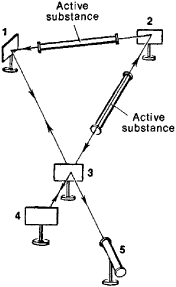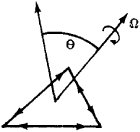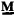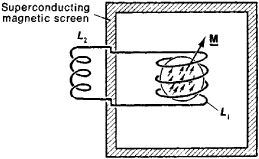Quantum Gyroscope

Also found in: Wikipedia.
The following article is from The Great Soviet Encyclopedia (1979). It might be outdated or ideologically biased.

Quantum Gyroscope

an instrument that is used to detect the rotation of a body and determine its angular velocity and is based on the gyroscopic properties of electrons, atomic nuclei, or photons.

Laser (optical) gyroscope. The sensing element of an optical gyroscope consists of a ring laser, which generates two light waves that propagate in opposite directions along a common light channel in the form of narrow, monochromatic beams. The resonator of the ring laser (Figure 1) consists of three or more mirrors (1), (2), and (3), mounted on a rigid support and forming a closed system. Part of the light passes through the semitrans-parent mirror (3) and impinges on the photoelectric detector (5). The wavelength generated by the ring laser (within the limits of the width of the spectral line of the working medium) is determined by the condition that a traveling wave must, in passing around the resonator contour, arrive at the starting point with its original phase. If the instrument is fixed, this condition is satisfied if the perimeter P of the contour accommodates a whole multiple n of the wavelength λ0 that is, if P = nλ0. In this case, the laser generates two opposing waves, whose frequency is identical and is equal to

V0 = c0 = cn/P

where c is the speed of light.

However, if the instrument rotates with angular velocity Ω about a direction subtending an angle θ to the perpendicular to its plane (Figure 2), the contour will rotate through a certain angle during the time required for the wave to travel around it. Depending on the direction of propagation of the wave, the path traversed by the wave will be less or more than P. Consequently, the frequencies of waves traveling in opposite directions become unequal. These frequencies v_ and v+ may be shown to beFigure 1. Diagram of a laser gyroscope: ( I ), (2), and (4) opaque mirrors; (3) sem~transparent mirror; (5) photoelectric detector

independent of the contour shape and are related to the rotation frequency Ω of the instrument by the equation

v± = v0 ± 2(v0/c)([SΩ. cos θ]/P)

where S is the area surrounded by the resonator contour. In this case the photoelectric detector, which is sensitive to light intensity, records the beats with a difference frequency

Δv = v+ − = kF cos θ

where F = Ω/2π and k = 8π(S/[λ0, P]). For example, for a square helium-neon quantum gyroscope with sides 25 cm long, λ0, = 6 × cm, leading to k = 2.5 × lo6. In this case, the rotation of the earth, which occurs with angular velocity π = 15 deg/hr at latitude θ = 60°, must lead to a beat frequency Δv = 15 Hz. If the axis of the quantum gyroscope is aimed at the sun, the latitude 9 of the location of the quantum gyroscope may be calculated from the beat frequency with an accuracy of up to a fraction of a degree, assuming that the earth's angular velocity of rotation Ω is known.Figure 2

Integration of the angular velocity of a rotating body with respect to time (which may be performed automatically) makes possible determination of the angle of rotation as a function of time. The sensitivity limit of the quantum gyroscope is theoretically determined by the spontaneous radiation of the atoms of the active medium of the laser. If an angle of rotation of 1 deg/hr corresponds to the beat frequency Δv = 1 Hz, then the limiting accuracy of the quantum gyroscope is equal to 10−3 deg/hr. This limit has not been attained by any means in existing quantum gyroscopes.

Nuclear and electron gyroscopes. Nuclear quantum gyroscopes use materials that have nuclear paramagnetism (water, organic liquids, gaseous helium, and mercury vapor). Atoms or molecules of such materials in the ground (unexcited) state have angular momentums caused only by the nuclear spin moments (the electron spin moments are compensated in these materials, because all electrons are paired). The magnetic moments of the nuclei are related to their spins. If the nuclear magnetic moments are oriented—for example, by means of an external magnetic field—and the orienting field is subsequently switched off, in the absence of other magnetic fields (for example, the earth’s magnetic field) the resulting total magnetic moment M will preserve its orientation in space for some time regardless of the change in orientation of the sensor. Such a static quantum gyroscope makes possible determination of the change in the position of a body connected to the sensor of the gyroscope.

Since the magnitude of the momentwill gradually decrease as a result of relaxation, materials with longer relaxation times are selected for quantum gyroscopes—for example, certain ororganic liquids, for which the relaxation time τ is several minutes, liquid 3He (about 1 hr), or a solution of liquid 3He in 4He (about 1 yr).

In a quantum gyroscope operating in the nuclear induction mode, rotation of the sensor, which contains nuclei with oriented magnetic moments, with angular velocity Ω is equivalent to the action of a magnetic field of intensity H = Ω/ϒnuc on the nuclei, where ϒnuc is the gyromagnetic ratio for the nuclei. Precession of the magnetic moments of the nuclei about the direction of the field H leads to the appearance of a variable electromotive force in the coil L, encompassing the working substance of the quantum gyroscope (Figure 3). Determination of the frequency of rotation Ω of the body connected with the quantum gyroscope’s sensor reduces to measurement of the frequency of the electric signal, which is proportional to Ω.Figure 3. Diagram of a nuclear positional gyroscope: () total magnetic moment of the substance, (L1) and (L2) induction coils

In the dynamic nuclear gyroscope, the total nuclear magnetic momentprecesses about the constant magnetic field H, which is rigidly connected to the device. Rotation of the sensor together with the field H with angular velocity Ω leads to a change in the precession frequency of the magnetic moment M that is approximately equal to the projection of the vector Ω on H. This change is recorded in the form of an electric signal. To attain high sensitivity and accuracy, such devices require high stability and uniformity of the magnetic field H. For example, for detection of the change in the precession frequency caused by the diurnal rotation of the earth, it is necessary that ΔH/H ≤ 10−9. The device is screened from the action of external magnetic fields by superconductors. For example, if the deflection of the sensor is due to the diurnal rotation of the earth, then the residual field in the screen should not exceed 3 × 10−9 oersted.

Electron quantum gyroscopes are analogous to the nuclear quantum gyroscopes, but materials are used in them that contain unpaired electrons in their atoms or molecules (for example, stable free radicals, or alkali metal atoms). Although the relaxation times of the electron spins are small, the use of such gyroscopes is promising, since the gyromagnetic ratio ϒel for electrons is several hundred times larger than for nuclei, and hence the precession frequency is greater, which is important for many applications.

Although quantum gyroscopes (particularly the optical types) are undergoing continuous improvement, their accuracy and sensitivity are still lower than in the best mechanical gyroscopes. However, quantum gyroscopes have a number of advantages over mechanical gyroscopes: they do not contain moving parts (they are inertialess), do not require arrestment, have a high degree of reliability and stability, may be started quickly, can withstand considerable acceleration, and are capable of operating at low temperatures. Some types of quantum gyroscopes are already being used not only as highly sensitive indicators of rotation, navigators, and gyrometers but also as gyrocompasses, surveying compasses, and sextants.

REFERENCES

Privalov, V. E., and S. A. Fridrikhov. “Kol’tsevoi gazovyi lazer.” Uspekhi fizicheskikh nauk, 1969, VOL. 97, ISSUE 3, P. 377.
Pomerantsev, N. M., and G. V. Skrotskii. “Fizicheskie osnovy kvantovoi giroskopii.” Uspekhi fizicheskikh nauk, 1970, VOL. 100, ISSUE 3, P. 361.

G. V. SKROTSKII